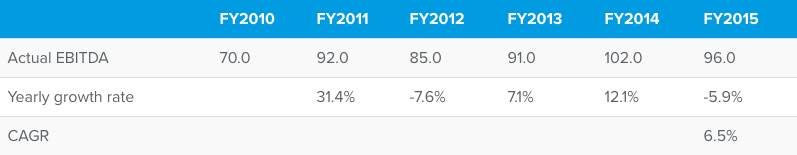5Y Historical EBITDA Growth is calculated as the compounded annual growth rate (CAGR) of EBITDA over the previous 5 financial years. Compounded annual growth rate is the average annual growth rate of an item over a specified period of time longer than one year. Consider the growth rates of EBITDA for a few years in the below table:While it is obvious that between FY2010 and FY2015 the EBITDA of the company increased, the increase and decrease in the interim years does not allow us to understand the approximate yearly growth rate. In such a scenario using compounded annual growth rate is very helpful. CAGR number in the above table has been calculated using the below formula:

((EBITDA in FY2015 / EBITDA in FY2010) ^ ( 1/N)) -1, where N is the number of years of growth rate i.e 5

Higher the historical EBITDA growth rate, the better.